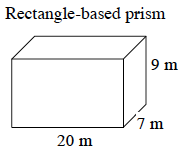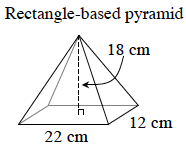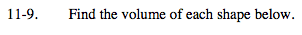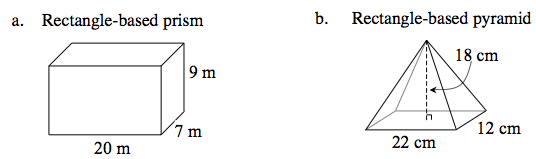Home > MC2 > Chapter 11 > Lesson 11.1.1 > Problem11-9

11-9.
1. Find the volume of each shape below. Homework Help ✎

1.2.Volume of a prism = (area of base)(height)

$\text{Volume of a pyramid} = \frac{1}{3} ( \text{area of base} )(\text{height})$(20m · 7 m)(9m) = 1260 m3